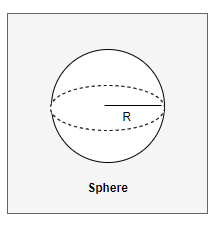# Sphere Calculator

Note
• Enter the values of Either Radius,Diameter,Volume,Area or Circumference
• Click on the calculate button to get the value of empty

Formula And concept used
if r is radius of the Sphere,then
$\text {Diameter} = 2r$
$\text {Surface Area}=4 \pi r^2$
$\text{Volume}= \frac {4}{3} \pi r^3$
$\text{circumference}= 2 \pi r$

## What is SphereSphere is a three dimensional geometricial shape.It is defined mathematically as the set of points that are all at the same distance r from a given point in a three-dimensional space. It is analogous to circle in two dimensional space.
The distance r is called the Radius of the Sphere.
Diameter of the Sphere is two times radius of the Sphere
$D= 2r$
Volume of the Sphere is given by the formula
$\text{Volume}= \frac {4}{3} \pi r^3$
Surface Area of the Sphere is given by the Formula
$\text {Surface Area}=4 \pi r^2$
Circumference of the greatest circle on the Sphere
$\text{circumference}= 2 \pi r$

Example of Few questions where you can use this Sphere Calculator
Question 1
Find the Surface Area of a Sphere whose radius is
i. 3 cm
ii. 10 cm
Solution
(i) r= 3cm
Surface Area of the Sphere is given by the Formula
$\text {Surface Area}=4 \pi r^2 = 4 \times 3.14 \times 3^2 = 113.04 \ cm^2$
(ii) r=10 cm
Surface Area of the Sphere is given by the Formula
$\text {Surface Area}=4 \pi r^2 = 4 \times 3.14 \times 10^2 = 1256 \ cm^2$

Question 2
Find the Volume of a Sphere whose radius is
i. 4 cm
ii. 12 cm
Solution
(i) r= 4cm
Volume of the Sphere is given by the formula
$\text{Volume}= \frac {4}{3} \pi r^3= \frac {4}{3} \times 3.14 \times 4^3 = 267.94 \ cm^3$
(ii) r=12 cm
Volume of the Sphere is given by the formula
$\text{Volume}= \frac {4}{3} \pi r^3= \frac {4}{3} \times 3.14 \times 12^3 = 7,234.56 \ cm^3$

Question 3
Find the Radius,Diameter, Volume and Circumference of a Sphere whose Surface area is 50.24 cm2
Solution
Given S=50.24 cm2, r=?, d=?, V=?, C=?
Surface Area of the Sphere is given by the Formula
$S=4 \pi r^2$
$r = \sqrt {\frac {S}{4 \pi }}= \sqrt {\frac {50.24}{4 \times 3.14 }} = 2 \ cm$
Now Volume of the Sphere is given by the formula
$\text{Volume}= \frac {4}{3} \pi r^3= \frac {4}{3} \times 3.14 \times 2^3 = 33.50 \ cm^3$
Diameter is given by
$d= 2 r = 2 \times 2 =4 \ cm$
Circumference of the greatest circle on the Sphere
$C= 2 \pi r= 2 \times 3.14 \times 2=12.56 \ cm$

## How Calculator works

• if radius of the Sphere(r) is given,then calculation done as below formula
$d = 2r$
$S=4 \pi r^2$
$V= \frac {4}{3} \pi r^3$
$C= 2 \pi r$
• if Diameter of the Sphere(d),then calculation done as below formula
$r = \frac {D}{2}$
$S=4 \pi r^2$
$V= \frac {4}{3} \pi r^3$
$C= 2 \pi r$
• if Surface Area of the Sphere(S),then calculation done as below formula
$r = \sqrt {\frac {S}{4 \pi }}$
$d=2r$
$V= \frac {4}{3} \pi r^3$
$C= 2 \pi r$
• if Circumference of the Sphere(C),then calculation done as below formula
$r = \frac {C}{2 \pi}$
$S=4 \pi r^2$
$V= \frac {4}{3} \pi r^3$
$d= 2 r$
• if Volume of the Sphere(V),then calculation done as below formula
$r = \sqrt  {\frac {3V}{4 \pi}}$
$S=4 \pi r^2$
$C= 2 \pi r$
$d= 2 r$